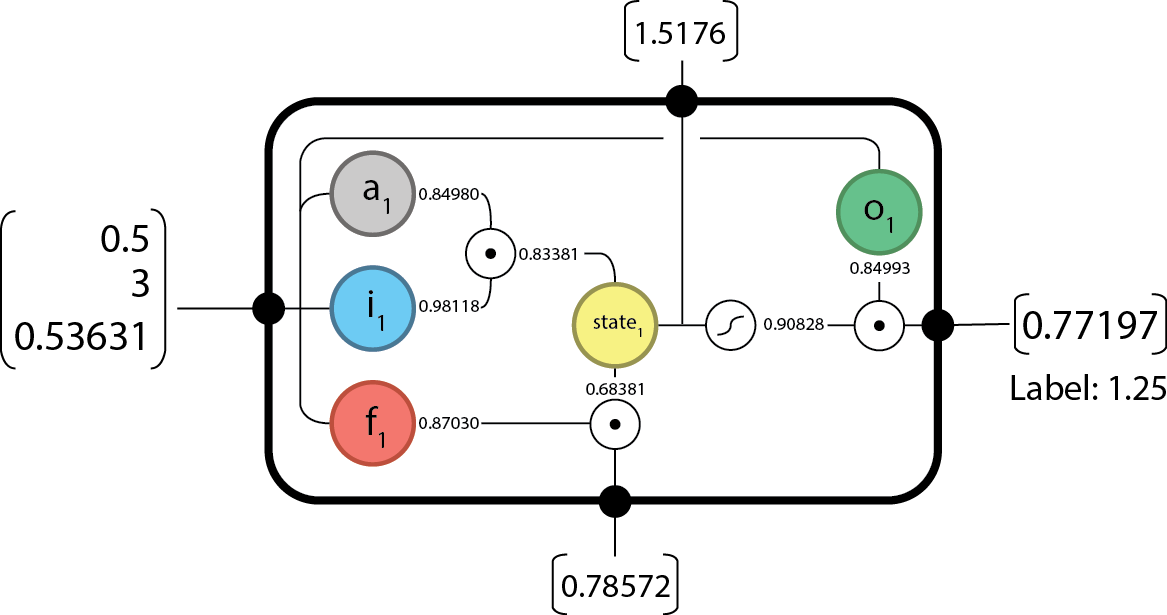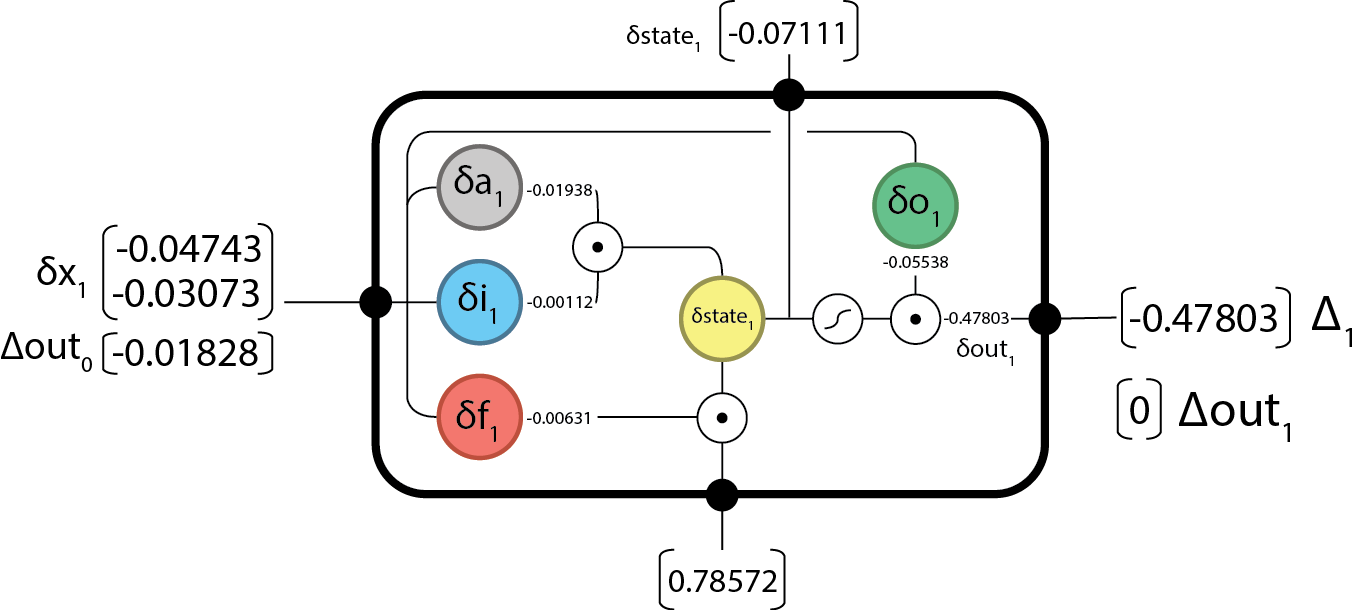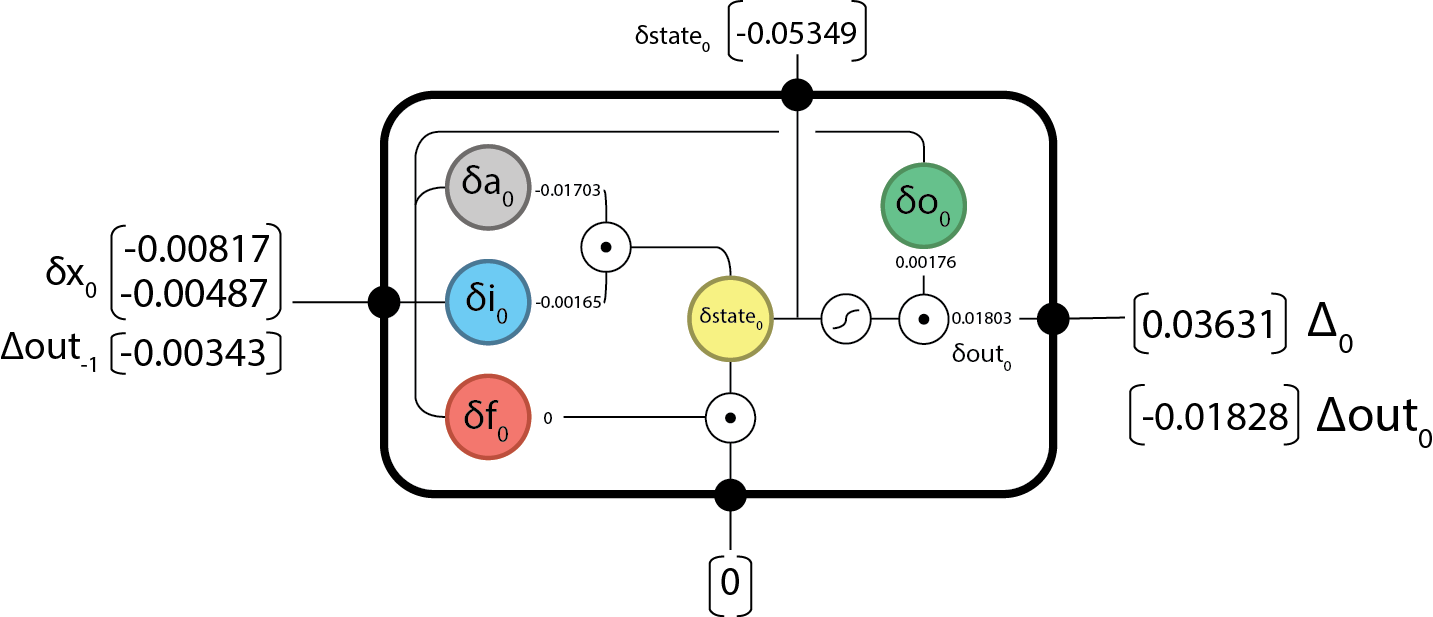# Backpropogating an LSTM: A Numerical Example

Let’s do this…

We all know LSTM’s are super powerful; So, we should know how they work and how to use them.## Syntactic notes

• Above $\bigodot$ is the element-wise product or Hadamard product.
• Inner products will be represented as $\cdot$
• Outer products will be respresented as $\bigotimes$
• $\sigma$ represents the sigmoid function: $\sigma(x) = \dfrac{1}{1 + e^{-x}}$

## The forward components

The gates are defined as:

• Input activation:
• Input gate:
• Forget gate:
• Output gate:

Note for simplicity we define:

• Internal state:
• Output:

## The backward components

Given:

• $\Delta_{t}$ the output difference as computed by any subsequent layers (i.e. the rest of your network), and;
• $\Delta out_{t}$ the output difference as computed by the next time-step LSTM (the equation for t-1 is below).

Find:

The final updates to the internal parameters is computed as:

Putting this all together we can begin…

# The Example

Let us begin by defining out internal weights:

And now input data:

I’m using a sequence length of two here to demonstrate the unrolling over time of RNNs

## Forward @ $t=0$From here, we can pass forward our state and output and begin the next time-step.

## Forward @ $t=1$And since we’re done our sequence we have everything we need to begin backpropogating.

## Backward @ $t=1$First we’ll need to compute the difference in output from the expected (label).

Note for this we’ll be using L2 Loss: $E(x, \hat x) = \dfrac{(x - \hat x)^{2}}{2}$. The derivate w.r.t. $x$ is $\partial_{x}E(x, \hat x) = x - \hat x$.

$\Delta out_{1} = 0$ because there are no future time-steps.

Now we can pass back our $\Delta out_{0}$ and continue on computing…

## Backward @ $t=0$And we’re done the backward step!

Now we’ll need to update our internal parameters according to whatever solving algorithm you’ve chosen. I’m going to use a simple Stochastic Gradient Descent (SGD) update with learning rate: $\lambda = 0.1$.

We’ll need to compute how much our weights are going to change by:

And updating out parameters based on the SGD update function: $W^{new} = W^{old} - \lambda * \delta W^{old}$ we get our new weight set:

And that completes one iteration of solving an LSTM cell!

Of course, this whole process is sequential in nature and a small error will render all subsequent calculations useless, so if you catch ANYTHING email me at hello@aidangomez.ca